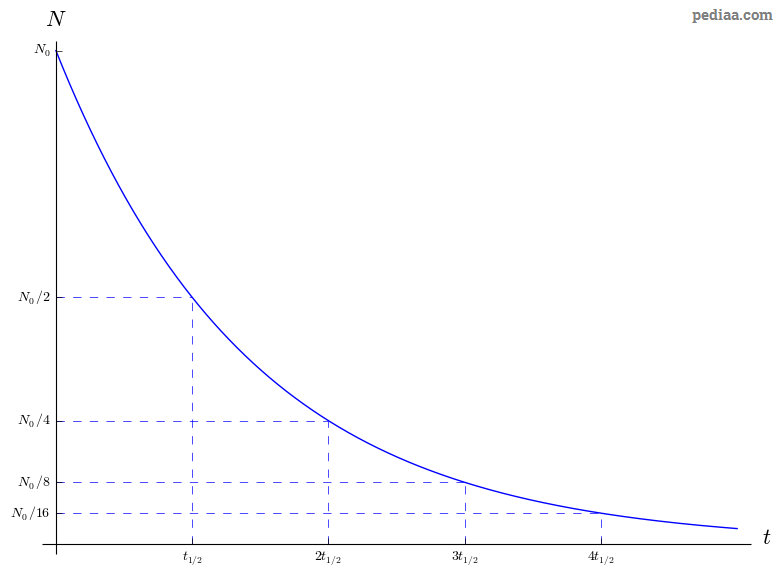# How to Calculate Half Life

In this section, we will learn about half life and derive the formula to calculate half life. In radioactivity, half life is the time taken by half of radioactive nuclei in a sample of a radioactive isotope to decay. The number of radioactive nuclei in a sample decay exponentially over time. To calculate half life, therefore, the mathematics of exponential decay is used. Half life is an extremely important concept for applications of radioactivity. Radioisotopes introduced to organs in radiotherapy, for instance, must not linger in a patient’s body for too long. On the other hand, isotopes used for dating historic artefacts must have long half lives so that enough of them have remained until the present day for us to determine the objects’ age.

## Difference Between Random and Spontaneous Nature of Radioactive Decay

Radioactive decay is categorised as both random and spontaneous.

• Radioactive decay is random because we cannot determine when a given nucleus will decay, or determine how long it would take before a given nucleus would decay. Consequently, each radioactive nucleus in a sample has the same probability for decaying at a given time.
• Radioactive decay is spontaneous because it is not affected by  external conditions.

## What is Half Life

The number of radioactive nuclei in a sample is decreasing, because once the nucleus decays through alpha, beta, and gamma decay they cannot undergo the same decay process again. The number of radioactive nuclei in the sample decreases exponentially.

The activity, or the decay rate, is the rate of change of the number of radioactive nuclei. This is given by,$-\frac{\mathrm{d}N}{\mathrm{d}t}=\lambda N$

The negative sign signifies that the number of radioactive nuclei in a sample is decreasing over time. $latex\lambda &s=1$ is called the decay constant. It gives the probability that a given nucleus will decay per unit time. The decay constant has a specific value for any given nuclear decay process.  The higher the$\lambda$, the higher the probability of decay and the number of radioactive nuclei in the sample diminishes quicker.

If the number of radioactive nuclei in a sample at a time$t=0$ is$N_0$, then the number of radioactive nuclei$N$ in the sample after a time$t$ is given by:$N(t)=N_0e^{-\lambda t}$

The number of radioactive nuclei in the sample decreases exponentially. Half life ($t_{1/2}$) is the amount of time taken for the number of radioactive nuclei in the time to become halved. If we draw a graph of how the number of radioactive nuclei in the sample vary over time, we get the following graph:How to Calculate Half Life – Radioactive Decay Curve

## How to Calculate Activity

Activity of the sample is proportional to the number of radioactive nuclei present. So, we can make an equivalent statement,$A(t)=A_0e^{-\lambda t}$

where$A(t)$ is the activity of the sample at time$t$, with$A_0$ the activity when$t=0$.

If a graph of activity vs. time is drawn, it will produce a graph with the same shape (i.e. activity also decays exponentially).

Activity is measured with the SI unit becquerel (Bq). An activity of 1 Bq corresponds to a rate of 1 decay per second. The curie (Ci) is another unit used to measure activity. 1 Ci = 3.7×1010 Bq.

## Half Life Formula

We will now derive a formula to get the half life from the decay constant. We start with,$N=N_oe^{-\lambda t}$

After a time$t=t_{1/2}$, the number of radioactive nuclei halves. So,$\frac{1}{2}N_0=N_oe^{-\lambda t_{1/2}}$, or$\frac{1}{2}=e^{-\lambda t_{1/2}}$

Taking the natural logarithm of both sides, we get:$-\mathrm{ln\:2}=-\lambda t_{1/2}$

and so,$t_{1/2}=\frac{\mathrm{ln\:2}}{\lambda}$

## How to Calculate Half Life

Example 1

Indium-112 has a half life of 14.4 minutes. A sample contains 1.32×1024 atoms of Indium-112.

a) Find the decay constant

b) Find out how many atoms of Indium-112 would be left in the sample after 1 hour.

a) Since$\lambda= \frac{\mathrm{ln\:2}}{t_{1/2}}$,$\lambda= \frac{\mathrm{ln\:2}}{14.4\times 60 \mathrm{\:s}}=8.02\times 10^{-4}\mathrm{\:s^{-1}}$

b) Using$N(t)=N_0e^{-\lambda t}$$N=1.32\times 10^{24}e^{-\left(8.02\times 10^{-4}\right)\times \left(60\times 60\right)}=7.35\times 10^{22}$ atoms.

Example 2

During a treatment for thyroid cancer, a patient is given a sample of Iodine-131 to ingest, which has an activity of 1.10 MBq. Half life of Iodine 131 is 8.02 days. Find the activity of iodine-131 in the patient’s body after 5 days of ingestion.

We use$A=A_0e^{-\lambda t}$. First, we work out$\lambda$:$\lambda=\frac{\mathrm{ln\:2}}{t_{1/2}}=\frac{\mathrm{ln\:2}}{8.02}=0.086{\mathrm {\:day^{-1}}}$

Then,$A=1.10e^{-0.086\times5}=0.71$ Mbq.

Note:

1. We directly calculated the decay constant per day and kept the half life also in days. So the days cancelled off when we calculated$\lambda t$ and there was no need to convert times to seconds (that would have worked as well, but it would have involved a little more calculation)
2. In reality, the activity would be smaller. This is because there is also a biological half life associated with the activity. This is the rate at which the patient excretes radioactive nuclei from their body.

Example 3

Calculate the half life of a radioactive isotope whose activity reduces by 4% over 1000 years.

4% = 0.04. We now have$\frac{A}{A_0}=0.04=e^{-\lambda\times 1000}$.  Taking ln of both sides,$-3.21=-\lambda\times 1000\Rightarrow \lambda =3.21\times 10^{-3}$ per year.$t_{1/2}=\frac{\mathrm{ln\:2}}{\lambda}=\frac{\mathrm{ln\:2}}{3.21\times 10^{-3}}=$ 216 years.